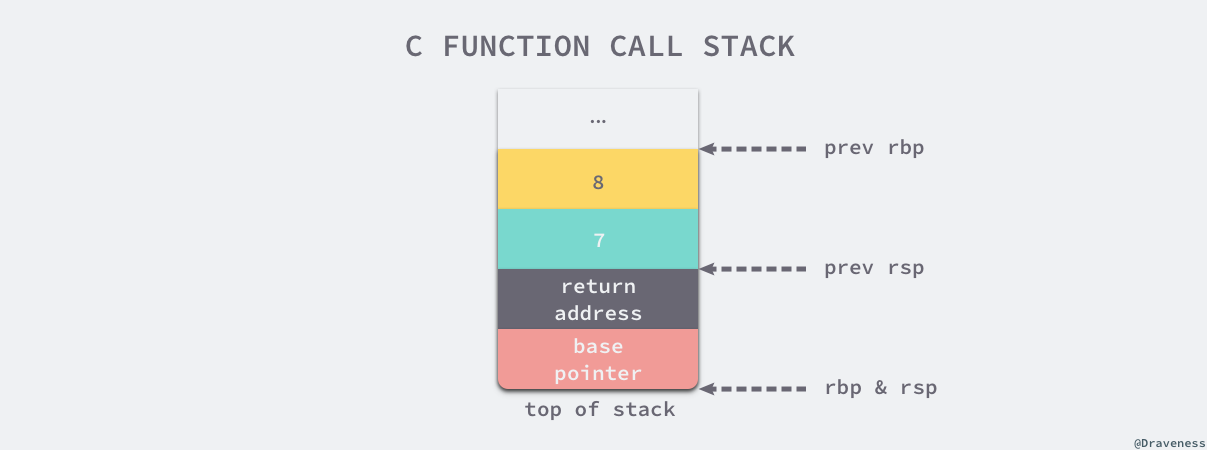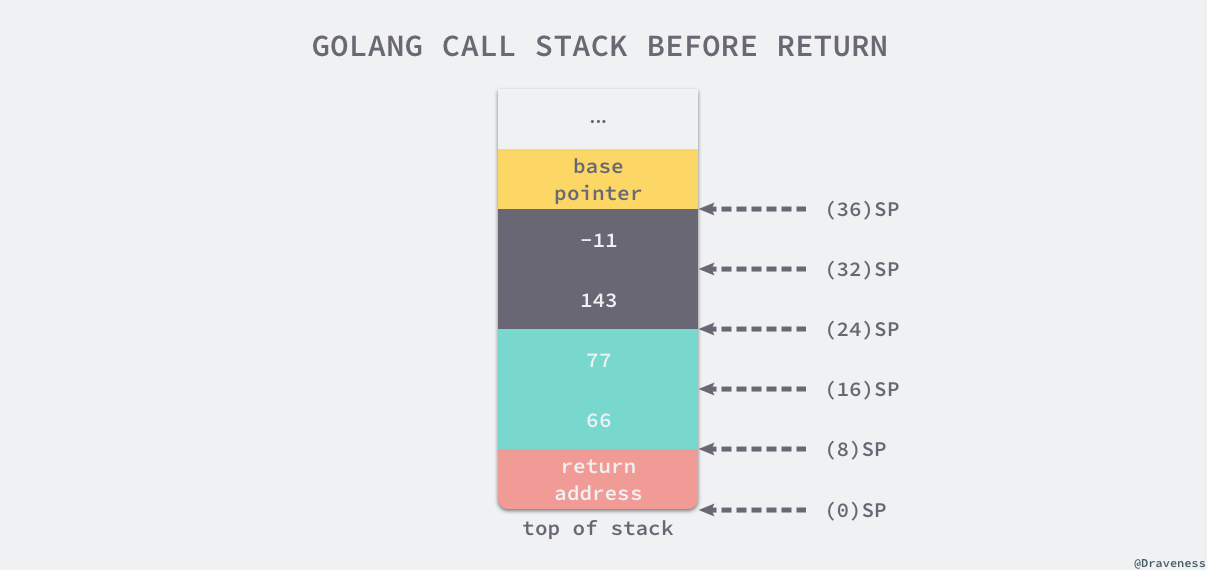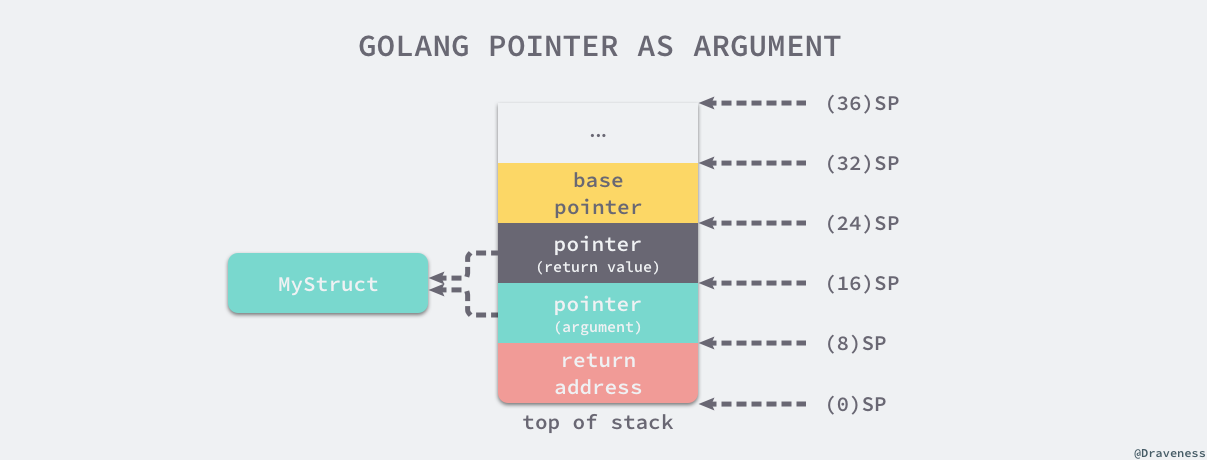# 2.1 函数调用 · 浅谈 Go 语言实现原理

Go to the source link to view the article. You can view the picture content, updated content and better typesetting reading experience. If the link is broken, please click the button below to view the snapshot at that time.

# 4.1 函数调用

## 4.1.1 调用惯例

``````somefunction(arg0, arg1)
``````

gcc 和 clang 编译相同 C 语言代码可能会生成不同的汇编指令，不过生成的代码在结构上不会有太大的区别，所以对只想理解调用惯例的人来说没有太多影响。作者在本节中选择使用 gcc 编译器来编译 C 语言：

``````\$ gcc --version
gcc (Ubuntu 4.8.2-19ubuntu1) 4.8.2
Copyright (C) 2013 Free Software Foundation, Inc.
This is free software; see the source for copying conditions.  There is NO
warranty; not even for MERCHANTABILITY or FITNESS FOR A PARTICULAR PURPOSE.
``````

``````// ch04/my_function.c
int my_function(int arg1, int arg2) {
return arg1 + arg2;
}

int main() {
int i = my_function(1, 2);
}
``````

``````main:
pushq	%rbp
movq	%rsp, %rbp
subq	\$16, %rsp
movl	\$2, %esi  // 设置第二个参数
movl	\$1, %edi  // 设置第一个参数
call	my_function
movl	%eax, -4(%rbp)
my_function:
pushq	%rbp
movq	%rsp, %rbp
movl	%edi, -4(%rbp)    // 取出第一个参数，放到栈上
movl	%esi, -8(%rbp)    // 取出第二个参数，放到栈上
movl	-8(%rbp), %eax    // eax = esi = 1
movl	-4(%rbp), %edx    // edx = edi = 2
addl	%edx, %eax        // eax = eax + edx = 1 + 2 = 3
popq	%rbp
``````

• `my_function` 调用前，调用方 `main` 函数将 `my_function` 的两个参数分别存到 edi 和 esi 寄存器中；
• `my_function` 调用时，它会将寄存器 edi 和 esi 中的数据存储到 eax 和 edx 两个寄存器中，随后通过汇编指令 `addl` 计算两个入参之和；
• `my_function` 调用后，使用寄存器 eax 传递返回值，`main` 函数将 `my_function` 的返回值存储到栈上的 `i` 变量中；
``````int my_function(int arg1, int arg2, int ... arg8) {
return arg1 + arg2 + ... + arg8;
}
``````

``````main:
pushq	%rbp
movq	%rsp, %rbp
subq	\$16, %rsp     // 为参数传递申请 16 字节的栈空间
movl	\$8, 8(%rsp)   // 传递第 8 个参数
movl	\$7, (%rsp)    // 传递第 7 个参数
movl	\$6, %r9d
movl	\$5, %r8d
movl	\$4, %ecx
movl	\$3, %edx
movl	\$2, %esi
movl	\$1, %edi
call	my_function
``````

`main` 函数调用 `my_function` 时，前六个参数会使用 edi、esi、edx、ecx、r8d 和 r9d 六个寄存器传递。寄存器的使用顺序也是调用惯例的一部分，函数的第一个参数一定会使用 edi 寄存器，第二个参数使用 esi 寄存器，以此类推。``````my_function:
pushq	%rbp
movq	%rsp, %rbp
movl	%edi, -4(%rbp)    // rbp-4 = edi = 1
movl	%esi, -8(%rbp)    // rbp-8 = esi = 2
...
movl	-8(%rbp), %eax    // eax = 2
movl	-4(%rbp), %edx    // edx = 1
addl	%eax, %edx        // edx = eax + edx = 3
...
movl	16(%rbp), %eax    // eax = 7
addl	%eax, %edx        // edx = eax + edx = 28
movl	24(%rbp), %eax    // eax = 8
addl	%edx, %eax        // edx = eax + edx = 36
popq	%rbp
``````

`my_function` 会先将寄存器中的全部数据转移到栈上，然后利用 eax 寄存器计算所有入参的和并返回结果。

• 六个以及六个以下的参数会按照顺序分别使用 edi、esi、edx、ecx、r8d 和 r9d 六个寄存器传递；
• 六个以上的参数会使用栈传递，函数的参数会以从右到左的顺序依次存入栈中；

``````package main

func myFunction(a, b int) (int, int) {
return a + b, a - b
}

func main() {
myFunction(66, 77)
}
``````

``````"".main STEXT size=68 args=0x0 locals=0x28
0x0000 00000 (main.go:7)	MOVQ	(TLS), CX
0x0009 00009 (main.go:7)	CMPQ	SP, 16(CX)
0x000d 00013 (main.go:7)	JLS	61
0x000f 00015 (main.go:7)	SUBQ	\$40, SP      // 分配 40 字节栈空间
0x0013 00019 (main.go:7)	MOVQ	BP, 32(SP)   // 将基址指针存储到栈上
0x0018 00024 (main.go:7)	LEAQ	32(SP), BP
0x001d 00029 (main.go:8)	MOVQ	\$66, (SP)    // 第一个参数
0x0025 00037 (main.go:8)	MOVQ	\$77, 8(SP)   // 第二个参数
0x002e 00046 (main.go:8)	CALL	"".myFunction(SB)
0x0033 00051 (main.go:9)	MOVQ	32(SP), BP
0x0038 00056 (main.go:9)	ADDQ	\$40, SP
0x003c 00060 (main.go:9)	RET
```````main` 函数通过 `SUBQ \$40, SP` 指令一共在栈上分配了 40 字节的内存空间：

SP+32 ~ BP8 字节`main` 函数的栈基址指针
SP+16 ~ SP+3216 字节函数 `myFunction` 的两个返回值
SP ~ SP+1616 字节函数 `myFunction` 的两个参数

`myFunction` 入参的压栈顺序和 C 语言一样，都是从右到左，即第一个参数 66 在栈顶的 SP ~ SP+8，第二个参数存储在 SP+8 ~ SP+16 的空间中。

``````"".myFunction STEXT nosplit size=49 args=0x20 locals=0x0
0x0000 00000 (main.go:3)	MOVQ	\$0, "".~r2+24(SP) // 初始化第一个返回值
0x0009 00009 (main.go:3)	MOVQ	\$0, "".~r3+32(SP) // 初始化第二个返回值
0x0012 00018 (main.go:4)	MOVQ	"".a+8(SP), AX    // AX = 66
0x0017 00023 (main.go:4)	ADDQ	"".b+16(SP), AX   // AX = AX + 77 = 143
0x001c 00028 (main.go:4)	MOVQ	AX, "".~r2+24(SP) // (24)SP = AX = 143
0x0021 00033 (main.go:4)	MOVQ	"".a+8(SP), AX    // AX = 66
0x0026 00038 (main.go:4)	SUBQ	"".b+16(SP), AX   // AX = AX - 77 = -11
0x002b 00043 (main.go:4)	MOVQ	AX, "".~r3+32(SP) // (32)SP = AX = -11
0x0030 00048 (main.go:4)	RET
```````myFunction` 返回后，`main` 函数会通过以下的指令来恢复栈基址指针并销毁已经失去作用的 40 字节栈内存：

``````    0x0033 00051 (main.go:9)    MOVQ    32(SP), BP
0x0038 00056 (main.go:9)    ADDQ    \$40, SP
0x003c 00060 (main.go:9)    RET
``````

C 语言和 Go 语言在设计函数的调用惯例时选择了不同的实现。C 语言同时使用寄存器和栈传递参数，使用 eax 寄存器传递返回值；而 Go 语言使用栈传递参数和返回值。我们可以对比一下这两种设计的优点和缺点：

• C 语言的方式能够极大地减少函数调用的额外开销，但是也增加了实现的复杂度；
• CPU 访问栈的开销比访问寄存器高几十倍3
• 需要单独处理函数参数过多的情况；
• Go 语言的方式能够降低实现的复杂度并支持多返回值，但是牺牲了函数调用的性能；
• 不需要考虑超过寄存器数量的参数应该如何传递；
• 不需要考虑不同架构上的寄存器差异；
• 函数入参和出参的内存空间需要在栈上进行分配；

Go 语言使用栈作为参数和返回值传递的方法是综合考虑后的设计，选择这种设计意味着编译器会更加简单、更容易维护。

## 4.1.2 参数传递

• 传值：函数调用时会对参数进行拷贝，被调用方和调用方两者持有不相关的两份数据；
• 传引用：函数调用时会传递参数的指针，被调用方和调用方两者持有相同的数据，任意一方做出的修改都会影响另一方。

### 整型和数组

``````func myFunction(i int, arr int) {
fmt.Printf("in my_funciton - i=(%d, %p) arr=(%v, %p)\n", i, &i, arr, &arr)
}

func main() {
i := 30
arr := int{66, 77}
fmt.Printf("before calling - i=(%d, %p) arr=(%v, %p)\n", i, &i, arr, &arr)
myFunction(i, arr)
fmt.Printf("after  calling - i=(%d, %p) arr=(%v, %p)\n", i, &i, arr, &arr)
}

\$ go run main.go
before calling - i=(30, 0xc00009a000) arr=([66 77], 0xc00009a010)
in my_funciton - i=(30, 0xc00009a008) arr=([66 77], 0xc00009a020)
after  calling - i=(30, 0xc00009a000) arr=([66 77], 0xc00009a010)
``````

``````func myFunction(i int, arr int) {
i = 29
arr = 88
fmt.Printf("in my_funciton - i=(%d, %p) arr=(%v, %p)\n", i, &i, arr, &arr)
}

\$ go run main.go
before calling - i=(30, 0xc000072008) arr=([66 77], 0xc000072010)
in my_funciton - i=(29, 0xc000072028) arr=([66 88], 0xc000072040)
after  calling - i=(30, 0xc000072008) arr=([66 77], 0xc000072010)
``````

### 结构体和指针

``````type MyStruct struct {
i int
}

func myFunction(a MyStruct, b *MyStruct) {
a.i = 31
b.i = 41
fmt.Printf("in my_function - a=(%d, %p) b=(%v, %p)\n", a, &a, b, &b)
}

func main() {
a := MyStruct{i: 30}
b := &MyStruct{i: 40}
fmt.Printf("before calling - a=(%d, %p) b=(%v, %p)\n", a, &a, b, &b)
myFunction(a, b)
fmt.Printf("after calling  - a=(%d, %p) b=(%v, %p)\n", a, &a, b, &b)
}

\$ go run main.go
before calling - a=({30}, 0xc000018178) b=(&{40}, 0xc00000c028)
in my_function - a=({31}, 0xc000018198) b=(&{41}, 0xc00000c038)
after calling  - a=({30}, 0xc000018178) b=(&{41}, 0xc00000c028)
``````

• 传递结构体时：会拷贝结构体中的全部内容；
• 传递结构体指针时：会拷贝结构体指针；

``````type MyStruct struct {
i int
j int
}

func myFunction(ms *MyStruct) {
ptr := unsafe.Pointer(ms)
for i := 0; i < 2; i++ {
c := (*int)(unsafe.Pointer((uintptr(ptr) + uintptr(8*i))))
*c += i + 1
fmt.Printf("[%p] %d\n", c, *c)
}
}

func main() {
a := &MyStruct{i: 40, j: 50}
myFunction(a)
fmt.Printf("[%p] %v\n", a, a)
}

\$ go run main.go
[0xc000018180] 41
[0xc000018188] 52
[0xc000018180] &{41 52}
``````

``````type MyStruct struct {
i int
j int
}

func myFunction(ms *MyStruct) *MyStruct {
return ms
}

\$ go tool compile -S -N -l main.go
"".myFunction STEXT nosplit size=20 args=0x10 locals=0x0
0x0000 00000 (main.go:8)	MOVQ	\$0, "".~r1+16(SP) // 初始化返回值
0x0009 00009 (main.go:9)	MOVQ	"".ms+8(SP), AX   // 复制引用
0x000e 00014 (main.go:9)	MOVQ	AX, "".~r1+16(SP) // 返回引用
0x0013 00019 (main.go:9)	RET
``````## 4.1.3 小结

1. 通过堆栈传递参数，入栈的顺序是从右到左，而参数的计算是从左到右；
2. 函数返回值通过堆栈传递并由调用者预先分配内存空间；
3. 调用函数时都是传值，接收方会对入参进行复制再计算；

## 4.1.4 延伸阅读

1. GCC, the GNU Compiler Collection https://gcc.gnu.org/ ↩︎

2. Clang: a C language family frontend for LLVM https://clang.llvm.org/ ↩︎

3. Understanding CPU caching and performance https://arstechnica.com/gadgets/2002/07/caching/2/ ↩︎